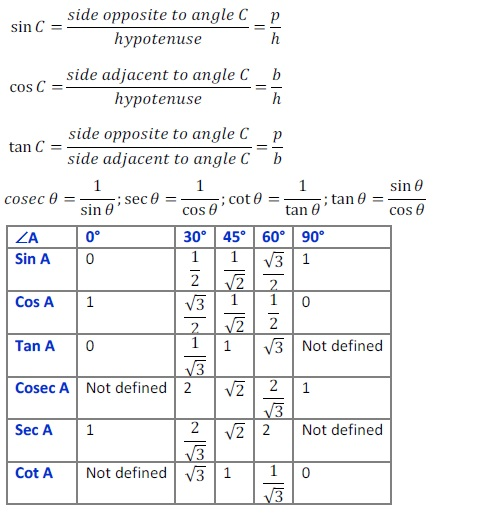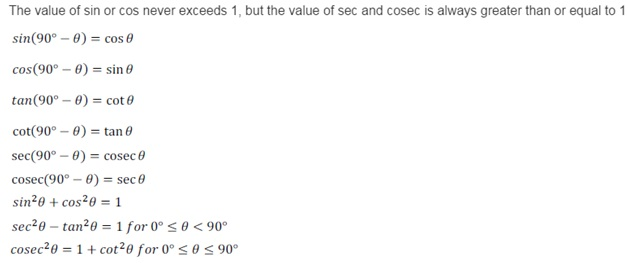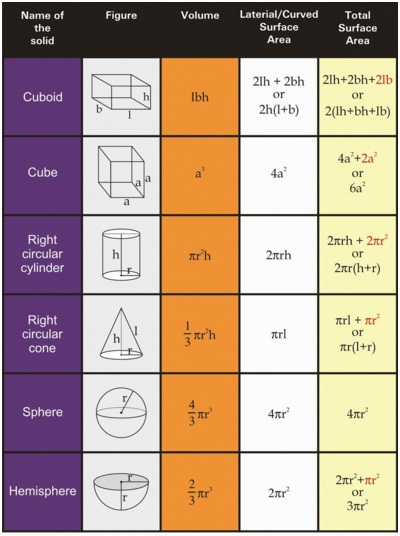# Important Maths Formulae For Class 10 CBSE

Most of the students are math phobic, they think it’s an extremely tough subject that they can’t master at all. This negative attitude towards their subject stops them from focusing on the math problems. They get nervous before exams even before a small class test as they are not at all prepared. To get rid from this situation, students need to hold a strong grip on this subject. If you hold it tightly, you can collect as many marks as you want. Understand the basic concepts, learn all the important formulae, apply formulae according to the concept and crack the most difficult question.

Here is a chapter-wise Marking scheme of Maths for class 10 that can help you prepare accordingly.

ChapterTopicsMarksAlgebraQuadratic Equations,Arithmetic ProgressionsMarks 26Geometry Marks 12Circles  Constructions Marks 22Trigonometry Marks 10Probability Marks 12Coordinate Geometry Marks 08Mensuration Marks 10 Total Marks 100

IMPORTANT MATHS FORMULAE

Algebra:

1. (a + b)2 = a2 + 2ab + b2
1. (a – b)2  = a2 – 2ab + b2
1. (a + b) (a – b) = a2 – b2
1. (x + a)(x + b)  = x2 + (a + b)x + ab
1. (x + a)(x – b)  = x2 + (a – b)x – ab
1. (x – a)(x + b)  = x2 + (b – a)x – ab
1. (x – a)(x – b)  = x2  – (a + b)x + ab
1. (a + b)3  = a3 + b3 + 3ab(a + b)
1. (a – b)3  =  a3 – b3 – 3ab(a – b)
1. (x + y + z) 2  = x2 + y2 + z2 + 2xy + 2yz + 2xz
1. (x + y – z) 2  =  x2 + y2 + z2 + 2xy – 2yz – 2xz
1. (x – y + z)2  = x2 + y2 + z2 – 2xy – 2yz + 2xz
1. (x – y – z)2  = x2 + y2 + z2 – 2xy +  2yz – 2xz
1. x3 + y3 + z3 – 3xyz  = (x + y + z)(x2 + y2 + z2 – xy – yz -xz)
1. x2 + y2  = 1212 [(x + y)2 + (x – y)2]
1. (x + a) (x + b) (x + c) = x3 + (a + b +c)x2 + (ab + bc + ca)x + abc
1. x3 + y3 = (x + y) (x2 – xy + y2)
1. x3 – y3  = (x – y) (x2 + xy + y2)
1. x2 + y2 + z2 -xy – yz – zx = 1212 [(x-y)2 + (y-z)2 + (z-x)2]

Powers:

amxan  = am+n

aman=am−naman=am−n

(am)n  =  amn

(ambn)p =   ampb np

a-m = 1am1am

amn=am−−−√namn=amn

Rules of Zero:

a1  = a

a0 = 1

a*0 = 0

a is undefined

Linear Equation:

Linear equation in one variable ax + b = 0, x = – −ba−ba

Quadratic Equation:  ax2 + bx + c  = 0  x  = −b±b2−4ac√2a−b±b2−4ac2a

Discriminant D = b2 – 4ac

Math Formulas:

When rate of discount is given Discount =   MP∗Rate of Discount 100

Simple Interest = PTR100PTR100 where P = Principal, T = Time in years R = Rate of interest per annum

Principal = 100∗S.IR∗T100∗S.IR∗T

Rate = 100∗S.IP∗T100∗S.IP∗T

Time = 100∗S.IP∗R100∗S.IP∗R

Principal = Amount – Simple Interest

Discount = MP – SP

Trigonometry:Probability

P(E) =   Number of outcomes favorable to E

Total Number of Possible Outcomes

Mensuration